Checkout JEE MAINS 2022 Question Paper Analysis : Checkout JEE MAINS 2022 Question Paper Analysis :

# Important Questions Class 8 Maths Chapter 6- Squares and Square Roots

Class 8 Maths important questions for chapter 6-Squares and Square Roots are available here with solutions. Our subject experts have also provided the solutions for these problems based on CBSE latest syllabus and in reference with NCERT book. Therefore, it will be easy for students to revise the chapter with this material and score in the final examination.

Students can also reach us at BYJU’S to get Maths subject important questions for class 8 for all the chapters. In chapter 6, we will learn to find the squares and square roots for different numbers.

Also, check:

## Important Questions with Solutions For Class 8 Maths Chapter 6 (Squares and Square Roots)

Q.1: How many numbers lie between squares of the following numbers?

(i) 12 and 13

(ii) 25 and 26

(iii) 99 and 100

Solution: As we know, between n2 and (n+1)2, the number of non–perfect square numbers are 2n.

(i) Between 122 and 132 there are 2×12 = 24 natural numbers.

(ii) Between 252 and 262 there are 2×25 = 50 natural numbers.

(iii) Between 992 and 1002 there are 2×99 =198 natural numbers.

Q.2: Write a Pythagorean triplet whose one member is:

(i) 6

(ii) 14

(iii) 16

(iv) 18

Solution:

We know, for any natural number m, 2m, m2–1, m2+1 is a Pythagorean triplet.

(i) 2m = 6

⇒ m = 6/2 = 3

m2–1= 32 – 1 = 9–1 = 8

m2+1= 32+1 = 9+1 = 10

Therefore, (6, 8, 10) is a Pythagorean triplet.

(ii) 2m = 14

⇒ m = 14/2 = 7

m2–1= 72–1 = 49–1 = 48

m2+1 = 72+1 = 49+1 = 50

Therefore, (14, 48, 50) is not a Pythagorean triplet.

(iii) 2m = 16

⇒ m = 16/2 = 8

m2–1 = 82–1 = 64–1 = 63

m2+ 1 = 82+1 = 64+1 = 65

Therefore, (16, 63, 65) is a Pythagorean triplet.

(iv) 2m = 18

⇒ m = 18/2 = 9

m2–1 = 92–1 = 81–1 = 80

m2+1 = 92+1 = 81+1 = 82

Therefore, (18, 80, 82) is a Pythagorean triplet.

Q.3: (n+1)2-n2 = ?

Solution:

(n+1)2-n2

= (n2 + 2n + 1) – n2

= 2n + 1

Q.4: Show that 121 is the sum of 11 odd natural numbers.

Solution: As 121 = 112

We know that the sum of first n odd natural numbers is n2.

Therefore, 121 = sum of first 11 odd natural numbers

= 1 + 3 + 5+ 7 + 9 + 11 +13 + 15 + 17 + 19 + 21

Q.5: Show that the sum of two consecutive natural numbers is 132.

Solution:

Let 2n + 1 = 13

So, n = 6

So, ( 2n + 1)2 = 4n2 + 4n + 1

= (2n2 + 2n) + (2n2 + 2n + 1)

Substitute n = 6,

(13)2 = ( 2 x 62 + 2 x 6) + (2 x 62 + 2 x 6 + 1)

= (72 + 12) + (72 + 12 + 1)

= 84 + 85

Q.6: Use the identity and find the square of 189.

(a – b)2 = a2 – 2ab + b2

Solution: 189 = (200 – 11)2

= 40000 – 2 x 200 x 11 + 112

= 40000 – 4400 + 121

= 35721

Q.7: What would be the square root of 625 using the identity (a +b)2 = a2 + b2 + 2ab?

Solution: (625)2

= (600 + 25)2

= 6002 + 2 x 600 x 25 +252

= 360000 + 30000 + 625

= 390625

Q.8: Find the square roots of 100 and 169 by the method of repeated subtraction.

Solution:

Let us find the square root of 100 first.

1. 100 – 1 = 99
2. 99 – 3 = 96
3. 96 – 5 = 91
4. 91 – 7 = 84
5. 84 – 9 = 75
6. 75 – 11 = 64
7. 64 – 13 = 51
8. 51 – 15 = 36
9. 36 – 17 = 19
10. 19 – 19 = 0

Here, we have performed a subtraction ten times.

Therefore, √100 = 10

Now, the square root of 169:

1. 169 – 1 = 168
2. 168 – 3 = 165
3. 165 – 5 = 160
4. 160 – 7 = 153
5. 153 – 9 = 144
6. 144 – 11 = 133
7. 133 – 13 = 120
8. 120 – 15 = 105
9. 105 – 17 = 88
10. 88 – 19 = 69
11. 69 – 21 = 48
12. 48 – 23 = 25
13. 25 – 25 = 0

Here, we have performed subtraction thirteen times.

Therefore, √169 = 13

Q.10: Find the square root of 729 using factorisation method.

Solution: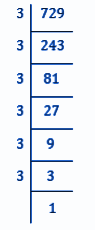729 = 3×3×3×3×3×3×1

⇒ 729 = (3×3)×(3×3)×(3×3)

⇒ 729 = (3×3×3)×(3×3×3)

⇒ 729 = (3×3×3)2

Therefore,

⇒ √729 = 3×3×3 = 27

Q. 11: Find the smallest whole number by which 1008 should be multiplied so as to get a perfect square number. Also, find the square root of the square number so obtained.

Solution:

Let us factorise the number 1008.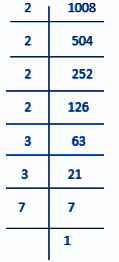1008 = 2×2×2×2×3×3×7

= (2×2)×(2×2)×(3×3)×7

Here, 7 cannot be paired.

Therefore, we will multiply 1008 by 7 to get a perfect square.

New number so obtained = 1008×7 = 7056

Now, let us find the square root of 7056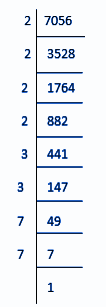7056 = 2×2×2×2×3×3×7×7

⇒ 7056 = (2×2)×(2×2)×(3×3)×(7×7)

⇒ 7056 = 22×22×32×72

⇒ 7056 = (2×2×3×7)2

Therefore;

⇒ √7056 = 2×2×3×7 = 84

Q. 12: Find the smallest whole number by which 2800 should be divided so as to get a perfect square.

Solution:

Let us first factorise the number 2800.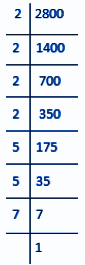2800 = 2×2×2×2×5×5×7

= (2×2)×(2×2)×(5×5)×7

Here, 7 cannot be paired.

Therefore, we will divide 2800 by 7 to get a perfect square.

New number = 2800 ÷ 7 = 400

Q. 13: 2025 plants are to be planted in a garden in such a way that each row contains as many plants as the number of rows. Find the number of rows and the number of plants in each row.

Solution:

Let the number of rows be, x.

Therefore, the number of plants in each row = x.

Total many contributed by all the students = x×x = x2

Given, x2 = Rs.2025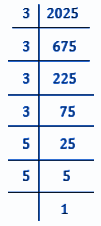x2 = 3×3×3×3×5×5

⇒ x2 = (3×3)×(3×3)×(5×5)

⇒ x2 = (3×3×5)×(3×3×5)

⇒ x2 = 45×45

⇒ x = √(45×45)

⇒ x = 45

Therefore,

Number of rows = 45

Number of plants in each rows = 45

Q.14: Find the smallest square number that is divisible by each of the numbers 8, 15 and 20.

Solution: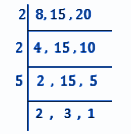L.C.M of 8, 15 and 20 is (2×2×5×2×3) = 120.

120 = 2×2×3×5×2 = (2×2)×3×5×2

Here, 3, 5 and 2 cannot be paired.

Therefore, we need to multiply 120 by (3×5×2) i.e. 30 to get a perfect square.

Hence, the smallest squared number which is divisible by numbers 8, 15 and 20 = 120×30 = 3600

Q.15: Find the square root of 7921 using long division method.

Solution: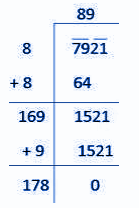∴ √7921 = 89

Q. 16: Find the square root of 42.25 using long division method.

Solution: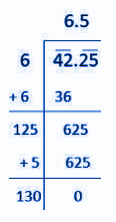∴ √42.25 = 6.5

Q. 17: Find the least number which must be added to 1750 so as to get a perfect square. Also, find the square root of the obtained number.

Solution:

Using long division method: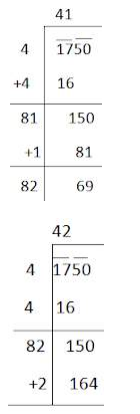Here, (41)2 < 1750 > (42)2

We can say 1750 is ( 164 – 150 ) = 14 less than (42)2.

Therefore, if we add 14 to 1750, it will be a perfect square.

New number = 1750 + 14 = 1764

Therefore, the square root of 1764 is as follows: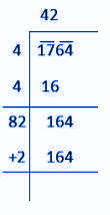∴√1764 = 42

### Class 8 Maths Chapter 6 Extra Questions

1. In a right triangle ABC, ∠B = 90°. a. If AB = 6 cm, BC = 8 cm, find AC b. If AC = 13 cm, BC = 5 cm, find AB.
2. Find the length of the side of a square whose area is 441 m2.
3. There are 500 children in a school. For a P.T. drill, they have to stand in such a manner that the number of rows is equal to the number of columns. How many children would be left out in this arrangement?
4. A gardener has 1000 plants. He wants to plant these in such a way that the number of rows and the number of columns remain the same. Find the minimum number of plants he needs more for this.
5. Express 49 as the sum of 7 odd numbers.
6. Find the square roots of the following decimal numbers
(i) 1056.25
(ii) 10020.01
7. Mention the smallest number, which when multiplied by 5408 gives a perfect square.

1. Nupur Mana Thorat

Good app 🙂

2. Sunny shah

This link helps my child to solve his dounghts THANK YOU very much _____BYJUS______to solve my childs dought

Good👍👍

Thanks it

5. Borde Seema Vithalrao

WOW,
I UNDERSTOOD EVERYTHING !!

6. Harshita

Great! Best site for exams. Can you give some fill ups type questions and mcqs approx. 20 each chapter? But without these questions, this is also perfect. Great effort. 👍👍👍👍

7. Very useful to me to prepare for my half yearly examsThanks a lot for this.

9. BHAVYA JAIN

It’s the best site for learning

10. SOUTIKAYAN

Nice website for exam preparation

11. Sidhdi Shendre

Very useful App Thank you Great effort
Now I can Understand Everything.

12. Harshita

It was a great revision for me to give my revision test on this chapter tomorrow

13. Sumeenash

It was a wonderful question which help my revision yesterday and the same came in my paper thank u 💘